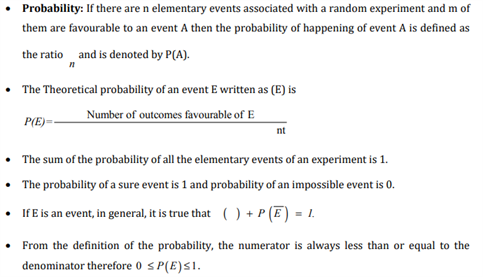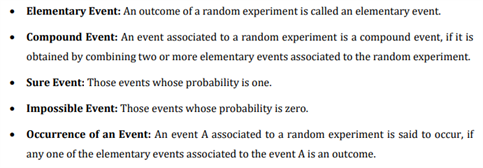# Revision Notes For CBSE Class 10 Maths Chapter 15 Probability

Probability is the final chapter and an important one given in class 10 maths textbook. In this chapter, students will be introduced to the theoretical probability of an event and simple problems based on this concept. However, the main aim of this chapter is to help students revise the basic rules of probability and clearly understand concepts like the Bayes’ theorem, multiplication rule of probability and independence of events. To help students feel comfortable with all the concepts in this chapter, CBSE revision notes for class 10 maths chapter 15 – probability is provided here. The notes can be downloaded freely and students can use it as a great reference tool to get insight into important topics, theories, and be well prepared to solve any question that can be asked in the examinations. More significantly, these notes will help students to stay ahead in the class.

Students can take a quick look of class 10 maths chapter 15 CBSE revision notes PDF below.Access CBSE Class 10 Maths Sample Papers Here.

Access NCERT Class 10 Maths Book Here.